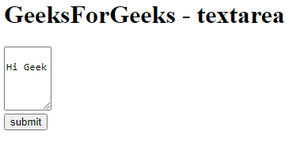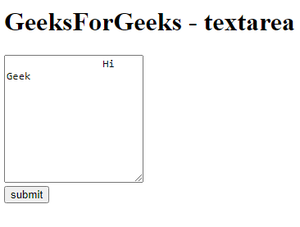Open in App
Not now

# Which tag can be used to control the editing for multi-line plain-text in HTML ?

• Last Updated : 01 Feb, 2022

In this article we will discuss the HTML tag that is used to control the editing for multi-line plain-text in HTML.

The <textarea> tag in HTML defines a multi-line plain-text editing control. It will be created with n rows and n columns. so we can say that the size of the text area will depend upon the number of rows and the number of columns.

Total textarea can be calculate using below formula:

`textarea = number_of_rows * number_of_columns`

Syntax:

`<textarea>Your_Text...</textarea>`

Example 1: In this example we are going to create a text area with 5 rows and 5 columns and a button named submit.

## HTML

 ` ` `<``html``> ` `<``body``> ` `    ``<``h1``>GeeksForGeeks - textarea ` `    ``<``form` `action``=``"#"``> ` `        ``<``textarea` `rows``=``"5"` `cols``=``"5"``> ` `        ``Hi Geek ` `        `` ` `        ``<``br``> ` `        ``<``input` `type``=``"submit"` `value``=``"submit"``> ` `    `` ` ` ` ``

Output:Example 2: In this example we are going to create a text area with 10 rows and 20 columns with name and required property set to true.

## HTML

 ` ` `<``html``> ` `<``body``> ` `    ``<``h1``>GeeksForGeeks - textarea ` `    ``<``form` `action``=``"#"``> ` `        ``<``textarea` `rows``=``"10"` `cols``=``"20"`  `                  ``name` `= ``"demo text"`  `                  ``required` `= ``"true"``> ` `        ``Hi Geek ` `        `` ` `        ``<``br``> ` `        ``<``input` `type``=``"submit"` `value``=``"submit"``> ` `    `` ` ` ` ``

Output:My Personal Notes arrow_drop_up
Related Articles